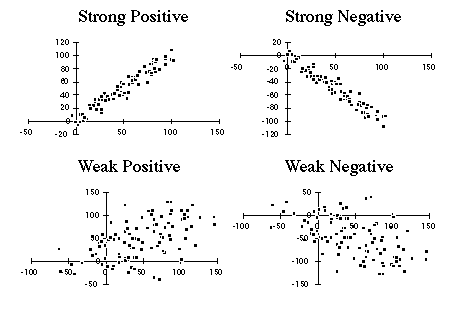# Identifying Correlated Data

Parameters that track each other are said to be correlated. If high values of one parameter tend to associated with high values of another parameter (and similarly, if low values of the first parameter are associated with low values of the second parameter), then the two parameters are said to be positively correlated.

On the other hand, if high values of the first parameter are associated with low values of the second parameter (and similarly, if low values of the first parameter are associated with high values of the second parameter), then the two parameters are said to be negatively correlated.

Correlations can be either strong or weak, depending upon the how reliably the two parameters track each other. The statistical parameter, r, is a measure of the degree of correlation, and varies from +1 for perfectly positively correlated parameters, thru 0, for uncorrelated data, to -1, for perfectly negatively correlated data.

Scatter plots are useful in identifying and displaying correlations, is in the figure, below.Note: The existence of a correlation does not necessarily mean that there is a cause and effect relationship between the two!

Note: These datasets were created by starting with a column of data containing the numbers (1, 2, 3 ... 100), and then making, and changing slightly, copies of this column. The positive correlations began with two copies of the column, to which random noise was added. The strong correlation has random noise of amplitude 5, and the weak correlation, 30. The negative correlations are just the data for the positive correlation, with the second column multiplied by -1.